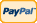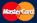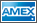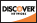•Electronics, electrical engineering
•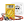Engineering physics
•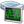Generators
•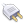Interfaces
•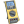Multimeters
•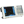Oscilloscopes
•Persona
•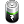Power supplies
•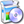Software
•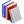Standards
•Thesaurus
•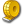Units of measurement
Filter by first letter
A
B
C
D
E
F
G
H
I
J
K
L
M
N
O
P
Q
R
S
T
U
V
W
X
Y
Z
+
-

# Mole (mol)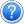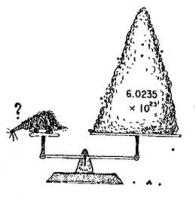The mole is a unit of measurement used in chemistry to express amounts of a chemical substance, defined as an amount of a substance that contains as many elementary entities (e.g., atoms, molecules, ions, electrons) as there are atoms in 12 grams of pure carbon-12 (12C), the isotope of carbon with atomic weight 12. This corresponds to a value of 6.02214179(30)×1023 elementary entities of that substance. It is one of the base units in the International System of Units, and has the unit symbol mol.

The mole is widely used in chemistry, instead of units of mass or volume, as a convenient way to express the amounts of reagents and products of chemical reactions.

The mole may also be used to express the number of atoms, ions, or other elementary entities in some sample. The concentration of a solution is commonly expressed by its molarity, the number of moles of the dissolved substance per liter of solution.

The number of molecules in a mole (known as Avogadro's number) is defined so that the mass of one mole of a substance, expressed in grams, is exactly equal to the substance's mean molecular weight.

Find more here: http://en.wikipedia.org/wiki/Mole_(unit).

Back to the list

Units Converter
• How it Works
• Company News
• Industry News & Events
• Measurement Fun Facts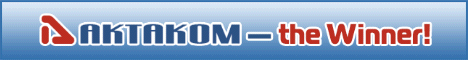Site map|Privacy policy|Terms of Use & Store Policies|How to Buy|Shipping|Payment|© T&M Atlantic, Inc., 2010-2022